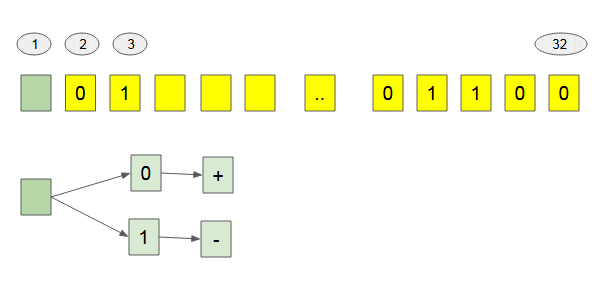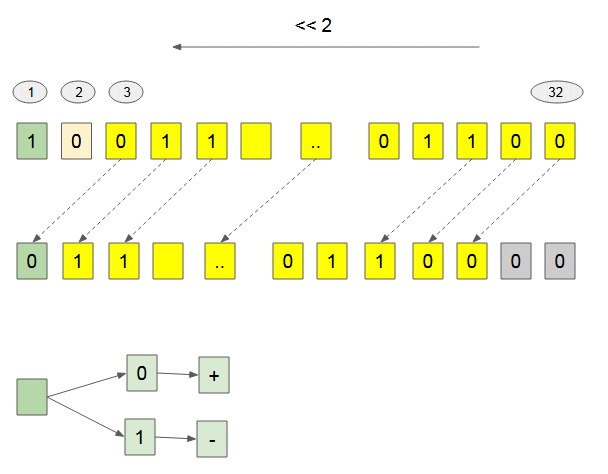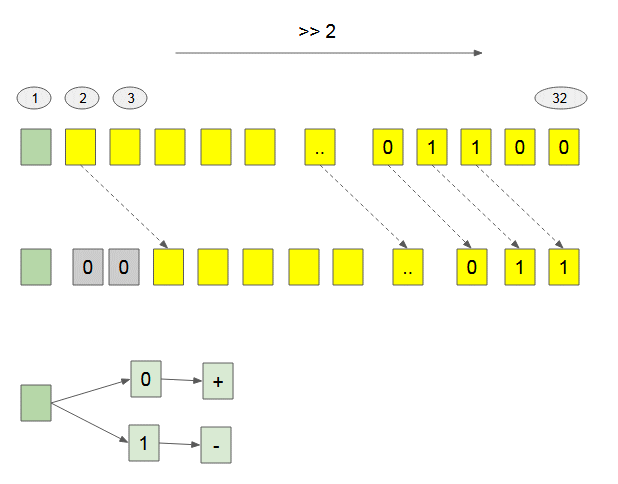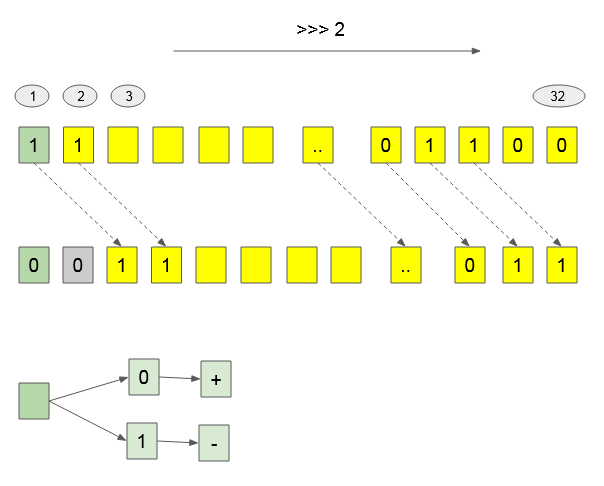# Bitwise Operations

## 1- Bitwise

In digital computer programming. The  bitwise operation works on one or more binary numerals, or binary numerals-like strings. This is a simple and fast operation, directly supported by  processor. Normally, bitwise operations are much faster than multiplication, division, sometimes significantly faster than addition. bitwise calculations use less energy because it rarely uses  resources.
There are 7 bitwise operations :
Operator Name Description
& AND If both bits are 1, the return value is 1, otherwise it returns 0.
| OR If one of two bits is 1, the return value is 1, otherwise it returns 0.
^ XOR If 2 bits are different, the return value is 1, otherwise it returns 0.
~ NOT Inverts all the bits, 0 to 1 and 1 to 0.
<< Zero fill left shift Shifts all bits to the left.
>> Signed right shift Shifts all bits to the right except the first bit.
>>> Zero fill right shift Shifts all bits to the right.

### Bitwise AND

When a bitwise AND is performed on a pair of bits, it returns 1 if both bits are 1, otherwise returns 0.
Example with 1 bit:
Operation Result
0 & 0 0
0 & 1 0
1 & 0 0
1 & 1 1
Example with 4 bit:
Operation Result
1111 & 0000 0000
1111 & 0001 0001
1111 & 0010 0010
1111 & 0100 0100

### Bitwise OR:

When a bitwise OR is performed on a pair of bits, it returns 1 if one of the bits are 1, otherwise returns 0.
Example with 1 bit:
Operation Result
0 | 0 0
0 | 1
1 | 0 1
1 | 1 1
Example with 4 bits:
Operation Result
1111 | 0000 1111
1111 | 0001 1111
1111 | 0010 1111
1111 | 0100 1111

### Bitwise XOR

When a bitwise XOR is performed on a pair of bits, it returns 1 if the bits are different, otherwise returns 0.
Example with 1 bit:
Operation Result
0 ^ 0 0
0 ^ 1
1 ^ 0 1
1 ^ 1
Example with 4 bits:
Operation Result
1111 ^ 0000 1111
1111 ^ 0001 1110
1111 ^ 0010 1101
1111 ^ 0100 1011

### Bitwise NOT

When a Bitwise NOT is used, it will reverse all bits, 1 to 0, and 0 to 1.
Example with 1 bit:
Operation Result
~ 0 1
~ 1 0
Example with 4 bits:
Operation Result
~ 0000 1111
~ 0001 0001
~ 0010 1101
~ 1100 0011

## 2- Bitwise operation on numbers

Different languages can have multiple data types to represent numbers.
• Java: byte, short, int, long, double.
• C#: byte, sbyte, short, ushort, int, uint, long, ulong, float, double
• Javascript: double
• .....
Javascript stores numbers as 64 bits floating point numbers. But bitwise operations are performed on 32- bit integers. Other languages such as Java, C#,.. bitwise operations are also performed on 32- bit integers.
Therefore, before carrying out the bitwise operations with numbers, you have to convert each number into a sequence of 32 binary numbers.
 Base 10 Base 2 32 bits 5 101 00000000000000000000000000000101 218 11011010 00000000000000000000000011011010
In  Javascript, the  toString(base) method helps you change any number from base 10 to other base.
toString-base-example.js (Javascript)
```
let a = 8;

// Base 2 string.
console.log( a.toString(2) );// 1000

// Base 8 string
console.log( a.toString(8) ); // 10

// Base 16 string
console.log( a.toString(16) ); // 8

let b = 218;

// Base 2 string.
console.log( b.toString(2) );// 11011010

// Base 8 string
console.log( b.toString(8) ); // 332

// Base 16 string
console.log( b.toString(16) ); // da

```
Example:
Decimal Binary
5 00000000000000000000000000000101
1 00000000000000000000000000000001
5 & 1 = 1 00000000000000000000000000000001
5 | 1  = 5 00000000000000000000000000000101
5 ^ 1  = 4 00000000000000000000000000000100
~ 5    = -6 11111111111111111111111111111010
Note: Of 32 bits, the first  bit is used to determine the sign of number. If this bit is 1, it corresponds to the ( - ) sign . If this  bit is 0 , it corresponds to the ( + ) sign### Bitwise Left Shift (<<)

Decimal Binary
5 00000000000000000000000000000101
5 << 1  = 10 00000000000000000000000000001010
5 << 2  = 20 00000000000000000000000000010100
The  Bitwise Left Shift ( << ) Operator can change the sign of number.(Javascript code)
```console.log( 1073741824 << 1); // -2147483648

console.log( 2684354560 << 1); // 1073741824
```

### Bitwise Right Shift (>>)  [Unsigned Right Shift]

Decimal Binary
29 00000000000000000000000000011101
29 >> 1  = 14 00000000000000000000000000001110
29 >> 2  = 7 00000000000000000000000000000111
The  Bitwise Right Shift ( >> ) operator doesn't change the sign of number because the first bit is not moved.### Bitwise Right Shift (>>>) [Zero fill right Shift]

The  Bitwise Right Shift ( >>> ) operator can change the number sign.(Javascript Code)
```console.log( -1073741824 >>> 1); // 1610612736

console.log( 1073741824 >>> 1); // 536870912
```

## 3- What is Bitwise used for?

The  bitwise calculations are directly supported by the computer processor, it performs very fast. Below, I list some examples of using these calculations.
Use the bitwise operations on  Strings (characters) in the  Java, C#, C/C++ ...languages. Note: some languages such as   Javascript  don't support the  bitwise operation with  String type.
Convert an Uppercase into Lowercase:
```// Rule: x | ' '

('A' | ' ') ==> 'a'
('B' | ' ') ==> 'b'

('a' | ' ') => 'a'
```
Convert Lowercase into Uppercase:
```// Rule: x & '_'

('a' & '_') ==> 'A'
('b' & '_') ==> 'B'

('A' & '_') ==> 'A'
```
Reverse uppercase to lowercase, lowercase to uppercase:
```// Rule: x ^ ' '

('a' ^ ' ') ==> 'A'
('A' ^ ' ') ==> 'a'
```
View the position of  Latin letters (applying to only uppercase)
```// Rule:  x & '?'

'A' & '?'  ==> 1
'B' & '?'  ==> 2
...
'Z' & '?'  ==> 26

// Rule:  x ^ '@'

'A' ^ '@'  ==> 1
'B' ^ '@'  ==> 2
...
'Z' ^ '@'  ==> 26

```
View the position of  Latin letters (applying only to lowercase)

```// Rule:  x ^ '`'

'a' ^ '`'  ==> 1
'b' ^ '`'  ==> 2
...
'z' ^ '`'  ==> 26

// '`' : binary ( 1100000 ), hex('60'),  chr(96)
```
View the position of Latin letters, applying to both uppercase and lowercase:
```// Rule:  x & '\u001F'

'A' & '\u001F'  ==> 1
'B' & '\u001F'  ==> 2
...
'Z' & '\u001F'  ==> 26

'A' & '\u001F'  ==> 1
'B' & '\u001F'  ==> 2
...
'Z' & '\u001F'  ==> 26

```